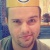• # Throwing Error

I would like to give some feedback about ...

HTTP_USER_AGENT:

```Mozilla/5.0 (Macintosh; Intel Mac OS X 10_12_0) AppleWebKit/537.36 (KHTML, like Gecko) Chrome/53.0.2785.143 Safari/537.36
```

I'm being able to run the code snippet both on devtools and node, the code gives the the correct answers in all tests, but when I try submitting it's not being accepted

```function hammingDistance(n, m){
n = (n).toString(2)
n = ("0".repeat(8 - n.length) + n).split("")
m = (m).toString(2)
m = ("0".repeat(8 - m.length) + m).split("")

var total = 0;

n.forEach((el, index) => {
if (el !== m[index]) {
total++;
}
})